Reach Us+44-1522-440391
Relative Likelihood Differences to Examine Asymptotic Convergen ce: A Bootstrap Simulation Approach | OMICS International
Journal of Biometrics & Biostatistics

Like us on:

All submissions of the EM system will be redirected to Online Manuscript Submission System. Authors are requested to submit articles directly to Online Manuscript Submission System of respective journal.

# Relative Likelihood Differences to Examine Asymptotic Convergen ce: A Bootstrap Simulation Approach

Milan Bimali* and Michael Brimacombe

Department of Biostatistics, University of Kansas Medical Center, Kansas City, 66160, KS, USA

*Corresponding Author:
Milan Bimali
MS, 3901 Rainbow Blvd
University of Kansas Medical Center
Kansas City, 66160
KS, USA
Tel: 913-588-4703
Fax: 913-588-0252
E-mail: [email protected]

Received date: February 05, 2015; Accepted date: April 25, 2015; Published date:May 05, 2015

Citation: Bimali M, Brimacombe M (2015) Relative Likelihood Differences to Examine Asymptotic Convergence: A Bootstrap Simulation Approach. J Biom Biostat 6: 220. doi:10.4172/2155-6180.1000220

Copyright: © 2015 Bimali M, et al. This is an open-access article distributed under the terms of the Creative Commons Attribution License, which permits unrestricted use, distribution, and reproduction in any medium, provided the original author and source are are credited.

Visit for more related articles at Journal of Biometrics & Biostatistics

#### Abstract

Maximum likelihood estimators (mle) and their large sample properties are extensively used in descriptive as well as inferential statistics. In the framework of large sample distribution of mle, it is important to know the relationship between the sample size and asymptotic convergence i.e. for what sample size does the mle behave satisfactorily attaining asymptotic normality. Previous works have discussed the undesirable impacts of using large sample approximations of the mles when such approximations do not hold. It has been argued that relative likelihood functions must be examined before making inferences based on mle. It was also demonstrated that transformation of mle can help achieve asymptotic normality with smaller sample sizes. Little has been explored regarding the appropriate sample size that would allow the mle achieve asymptotic normality from relative likelihood perspective directly. Our work proposes bootstrap/simulation based approach in examining the relationship between sample size and asymptotic behaviors of mle. We propose two measures of the convergence of observed relative likelihood function to the asymptotic relative likelihood functions namely: differences in areas and dissimilarity in shape between the two relative likelihood functions. These two measures were applied to datasets from literatures as well as simulated datasets.

#### Keywords

Relative likelihood functions; Bootstrap; Sample size; Divergence; Exponential family; Convergence

#### Introduction

“Likelihood” is arguably the most pronounced terminology in the statistical realm and was defined and popularized by the eminent geneticist and statistician Fisher [1-4]. The likelihood function is a function of model parameter(s) based on a given set of data and a predefined probability density function (pdf). The Likelihood function is formally defined as follows:

If f(x/θ) is the joint pdf (pmf) of the sample x=(x1,…,xn) with X' i s iid ,then the likelihood function of θ is given by: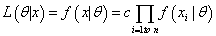Where c is a constant with respect to θ.

A key point often reiterated in textbooks is that the likelihood function is a function of θ and not to be viewed as a probability density itself . However, the shape of the likelihood function relative to its mode is often of interest in estimating θ. Likelihood functions can be mathematically constructed for most statistical distributions; however maximum likelihood estimators may not always have closed form . Nevertheless most of the distributions commonly used allow the computation of maximum likelihood estimators either analytically, numerically or graphically. Several properties of maximum likelihood estimators such as asymptotic normality, invariance, and ease of computation have made maximum likelihood estimators popular . In this paper we assume θ is a scalar throughout.

The large sample distribution of the maximum likelihood estimators is often used for inferential purposes. If θ is the mle of θ, then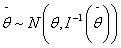where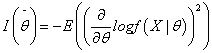is the Fisher Information evaluated at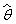. In situations where the computation of the expectation for the Hessian of the log-likelihood is analytically tractable,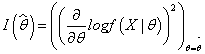the observed Fisher Information, has been used as an approximation in computation of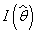.

A common question that often arises in statistics is in regard to sample size. In the framework of the large sample distribution of the mle, we are interested in knowing for what sample size the mle behaves satisfactorily, attaining the asymptotic normal distribution. Put a different way, does the existing sample size allow us to use the large sample properties of the mle with confidence? If not, what would be an ideal sample size?

Sprott et al. have elicited some of the undesirable impacts of using large sample approximation of the mle when such approximations do not seem to hold . They argue in favor of examining likelihood functions before making inferences about mle. They demonstrate via an example from Bartholomew  that drawing inferences from the mle without first examining the likelihood functions can be misleading. Figure 1 gives the plot of the relative likelihood (likelihood functions scaled by their mode) as obtained from Bartholomew’s data and relative normal likelihood (likelihood functions scaled by their mode) based on the large sample theory of mle. The plot shows that for a pre-specified value of relative likelihood, the range of θ s can be in complete disagreement between the two likelihood functions. E.g. for relative likelihood of 10% or higher, the ranges are roughly (20,110) and (7.81) for the relative and relative normal likelihood function , approximately 17% drop in coverage. Sprott et al. also demonstrated that transformation of the mle can help achieve the asymptotic normality with smaller sample sizes. However little has been explored regarding the appropriate sample size that would allow the mle to achieve asymptotic normality from a relative likelihood perspective directly (Figure 1). This work proposes a bootstrap/simulation based approach to the above question via the behavior and properties of relative likelihood function. In particular we measure the proximity of the observed likelihood function based on the actual sample to the likelihood function based on large sample properties, both of which are scaled here by their modes to have a maximum at one. The two convergence measures proposed by the authors are (i) difference in area under the two relative likelihood functions and (ii) dissimilarity in the shape of the two likelihood functions (dissimilarity index). We propose that, for a given sample size, if the difference in the area under the two relative likelihood functions and the dissimilarity index between them are both close to 0, the asymptotic approximation of mle is satisfactorily achieved. To study the properties of these measures and related likelihood convergence, we use the bootstrap to generate samples of varying size based on initial samples for examples in literature.

Figure 1: Observed Relative and Asymptotic Relative Likelihood Functions for Bartholomew’s Data.

The paper is laid out as follows. Section 2 provides a review of bootstrap method and some of the proposed measures of distance between distributions. In section 3, we provide the mathematical details of the two measures of convergence. In section 4 we provide examples by simulating data from exponential families of distribution and apply our method to some of the data available in literature and textbook.

Review of Bootstrap and Distances between Distributions

Bootstrap

The Bootstrap is a re sampling technique introduced by Efron with a related long history  and has attracted immense attention in the past three decades primarily due to its conceptual simplicity and due to the computational empowerment of statisticians due to advances in computer science . The past three decades have witnessed numerous works dedicated to developing bootstrap methods [13-19]. Bootstrap at its core, is a re sampling technique that treats the data at hand as a “surrogate population” and allows re sampling with replacement with a goal of re-computing the statistic of interest many times. This allows us to examine its distribution. Efron has demonstrated that bootstrap method outperforms other re sampling methods such as jackknifing and cross-validation . The distribution of the computed statistics is referred to as the bootstrap distribution. Despite the mathematical modesty of bootstrap algorithm, the large sample properties of bootstrapping distributions are surprisingly elegant. Singh, for example has demonstrated that the sampling distribution of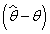, where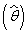is an estimate of θ, is approximated well enough by its bootstrap distribution . Bickel and Freedman have also made substantial contributions in developing bootstrap theory [21-23]. The most common applications of the bootstrap in its basic form involve approximating standard error of sample estimate, correcting the bias in the sample estimate, and in constructing confidence intervals. However in situations involving bootstrapping dependent data, modified bootstrap approaches such as moving-block bootstrap are recommended . Romano has discussed extensively the applications of bootstrap .

Distance between distributions

Kullback-Leibler distance is a commonly used measure of difference between two statistical distributions . If p(x) and q(x) are two continuous distributions the KL distance between p(x) and q(x) is defined as follows: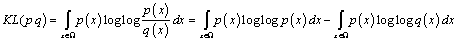Kullback-Leibler distance has been applied in areas such as functional linear model, Markovian process, model selection, and classification analysis [26-29]. It should be noted that the Kullback- Leibler distance is not symmetric,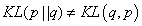, but can be expressed in a symmetric form .

Bhattacharya distance is another popular measure of difference between two distributions . If p(x) and q(x) are two continuous distributions the Bhattacharya distance between p(x) and q(x) is defined as follows: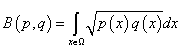The measure for discrete distribution is identical with integral replaced by summation. Bhattacharya distance has also found extensive applications in several fields [32-35]. Bhattacharya distance assumes the product p(x) q(x) to be non-negative.

In lieu of the above two distance measures, we could simply use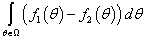as a measure of proximity between the two functions f1(θ) and f2(θ). Geometrically this measure is the difference in the area under the two curves generated by f1(θ) and f2(θ).

In this paper we make use of the bootstrap approach to resample from the actual sample (or simulate data from known distributions) to obtain a “bootstrap sample”. The size of the re sampled “bootstrap sample” is taken to exceed the size of actual sample. For each “bootstrap sample”, the observed relative likelihood function and corresponding asymptotic (normal) relative likelihood function are constructed and the area under the two relative likelihood functions computed. As the size of “bootstrap sample” increases we measure the convergence of the observed relative likelihood function to the asymptotic relative likelihood function. The convergence is measured by the difference in area under the curves and a dot product based measure of curve similarity. We note that simulated data is not a real world data and the sample sizes determined here are obtained in an ideal situation.

#### Method

Background

Let x=(x1,…,xn) be iid random variables from a specified distribution f(x/θ) with observed values x=(x1,…,xn). The observed relative likelihood function of θ i.e. R(θ) is defined as follows: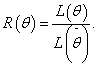Since, the asymptotic relative (large sample normal) likelihood function of θ can be defined as follows: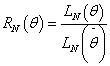For exponential families, the density function can be expressed in the following form: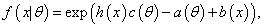and the likelihood function can be expressed as: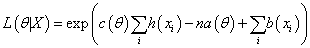Ifis the mle of θ, then the likelihood function evaluated atis: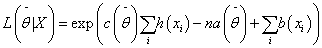Thus the observed relative likelihood function R(θ) is: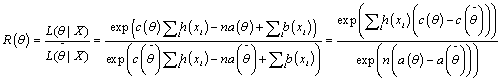The asymptotic relative likelihood function foris normally distributed and after scaling it by its value at mle, it assumes the following form: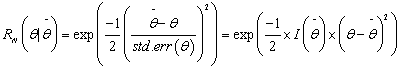where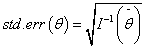andevaluated at.

In situations where computation of expectations is not analytically tractable,will be estimated by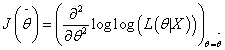.Here both R(θ) and RN(θ) are positive since both are exponential functions.

Measure of distance between R(θ) and RN(θ)

If R(θ) and RN(θ) are defined over the intervalL θU), the difference in area under the two likelihood curves will serve as the measure of discrepancy between R(θ) and RN(θ) and can be computed analytically as follows: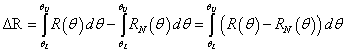If the expression does not have a closed form solution, numerical methods such as Simpson’s rule  can be applied: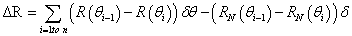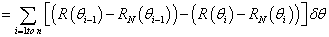Where n is the number of intervals.

For similar curves we would expect ΔR to be very small. “How small is small?” - the examples in the next section demonstrate that different distributions have different thresholds. This is primarily related to the fact that the domain of the parameters varies for different distributions. For example, in binomial distribution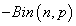,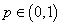whereas in the exponential distribution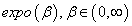. It is thus recommended that the measure of proximity should be considered on case by case basis. A tolerance level may also bet set: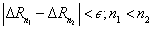are sample sizes. Typically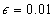will be acceptable.

Property of ΔR

1. On a log scale, R(θ/X) can be approximated by RN(θ/X) up to a second term .

Proof:

The general expression for Taylor expansion of a function f(x) around ‘a’ is as follows: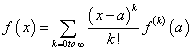where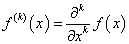Using Taylor expansion on log(R(θ/X)) aroundwe have: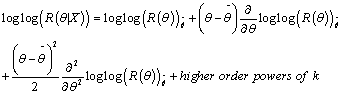Now: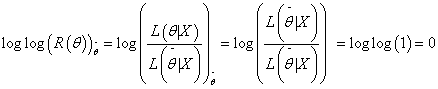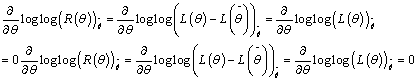This is the derivative of the score function evaluated at mle.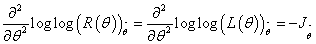Thus loglog(R(θ/X)) can be approximated as: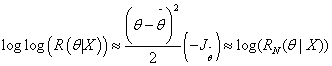The k! in the higher order terms of the Taylor expansion shrinks it to 0.

For exponential families: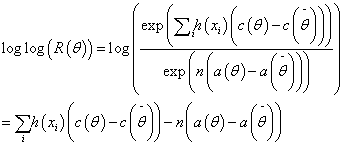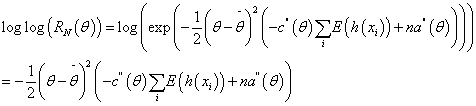So,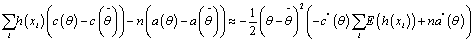This implies that higher order terms in the Taylor expansion areconverging to zero. Our method here graphically demonstrates this asa function of n.

Curve dissimilarity index

Let L1(θ) and L2 (θ) be two different functions of θ with the samedomain Ω. GraphicallyL1(θ) and L2 (θ) can be visualized as twocurves constructed on the same support. The two curves need notnecessarily have closed functional form. Here we propose a simpleand computationally efficient algorithm that uses the dot product tomeasure the similarity of the two curves in terms of their curvature.

The idea is to divide the support of the two curves into sufficientlysmall segments so that each of them can be approximated by a linesegment (Figure 2). Each of these segments is equivalent to a vectorin two dimensions and hence we can compute the dot product for thetwo vectors in each of these segments. If in general the two vectors areparallel in each of these segments, this would imply that the two curveshave similar local curvature and hence the curves are locally similar.In other words, for similar curves, the dot product between the twovectors is equal to the product of their individual L2 norms over eachsegment (Figure 2).

Let i=1,…,n+1, be the number of points over which the two curvesare segmented i.e. there are n segments of the two curves in total. S1i(θ)and S2i (θ) be any segment of the two curves.

Let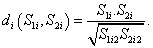(2)

Properties of di: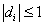di=1 if S1i and S2iare parallel. This is the case for perfect similarity.

di=1 if S1i and S2i are in opposite direction. This is the case forperfect dissimilarity.

Ideally if two curves were exactly same, we would expect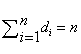(3)

A Dissimilarity index

Equation (3) can be used to express disagreement between the twocurves (here referred to as dissimilarity index). If D is the dissimilarityindex between the two curves then,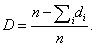Note that: 0 ≤ ≤1.

The bootstrap algorithm

The proposed bootstrap algorithm can be summarized in thefollowing steps.

1. For a given sample x=(x1,…,xn), compute R(θ) and RN(θ).

2. Choose tolerance level for ΔR and D.

3. Compute ΔR and D for the given sample.

If ΔR and D are not sufficiently close to 0, bootstrap from theoriginal sample and compute ΔR and D again for the bootstrappedsample.

Repeat step 3 until satisfactory convergence in achieved i.e. D andΔR is less than chosen tolerance level.

The next section contains several simulated examples todemonstrate the application of the above method.

#### Results

In this section, we examine the convergence of likelihood functions for some of the common distributions, using simulated data as well as for data obtained from the literature. Expression for R(θ) and RN(θ)for some common distributions are tabulated in Table 1. We would like to reiterate that R(θ) and RN(θ) are the observed and large sample normal likelihood functions scaled by their modes.

Distribution R(θ) RN(θ) Note
Poisson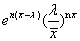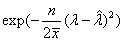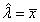Binomial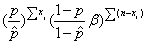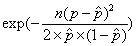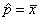Exponential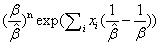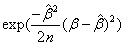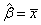Weibull(Shape parameter fixed)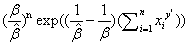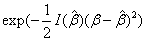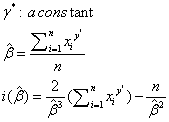Table 1: Relative and relative Normal likelihood functions for some exponential families Observed and asymptomatic relative like hood functions for some distributions in exponential families.

Simulation studies

The convergence of the observed relative likelihood function to asymptotic relative likelihood function was first examined using simulated dataset. For different families of exponential distributions, data were simulated for a given sample size. For the given data, the two convergence measures namely ΔR and D were computed. This process was repeated for different sample sizes and the values ofΔR and D thus obtained were recorded. The examples of some of the distributions from exponential families and the required sample sizes that makes the large sample approximation of the mle reasonable are presented in Tables 1 and 2 and in Figures 3-6. Additional examples for moredistributions from exponential families are provided in supplementary materials.

n ∆R:Difference in Area D:Dissimilarity Index
1 0.0691 0.01968
3 0.01318 0.01183
5 0.00604 0.00767
7 0.00359 0.005752
10 0.00201 0.00374
15 0.00114 0.00303

Table 2: Poisson Distribution: Values of difference in area and dissimilarity index for data of different sample sizes simulated from Poisson distribution.

Example 1: Poisson distribution (λ=10)

Example 2: Wei bull Distribution (γ=2, β=6)

Examples Using Data from Literature

a) Data from Gibbons et al.’s book “Nonparametric Statistical Inference” 

A group of 20 mice are allocated to individual cages randomly. The cages are then assigned randomly to two treatments namely control A and drug B. All animals were infected with tuberculosis. The number of days until the mice die is recorded (Tables 3 and 4).

n ∆R:Differnce in Area D:Dissimilarity Index
10 0.35706 0.34744
15 0.20736 0.23947
30 0.08872 0.16687
50 0.04498 0.10846
75 0.02406 0.07855
100 0.0158 0.0615

Table 3: Weibull Distribution Values of difference in area and dissimilarity index for data of different sample sizes simulated from Weibull distribution.

Number of days untill death Mean Variance
control 5,6,7,7,8,8,8,9,12 7.778 3.944
drug 7,8,8,8,9,12,1,3,14,17 10.5 10.944

Table 4: Data from Gibbon’s et al.Values represent number of days each mice survived when exposed to control or drug.

For mice assigned to drug the mean and variance are roughly equal and the data is count data. So a Poisson model is a reasonable choice. Based on the proposed methods, the values of difference in area under curves ΔA and dissimilarity index were found to be D: 0.00204 and 0.0066 respectively. It indicates that the asymptotic normality approximation of the mles holds for the data (Drug) above (Figure 7).

The following data was obtained from Williams et al. [39,40]. The data is the weight (in grams) of dry seed in the stomach of each spinifex pigeon captured in desert. The data is as follows:

0.457, 3.751, 0.238, 2.967, 2.509, 1.384, 1.454, 0.818, 0.335, 1.436, 1.603, 1.309, 0.201, 0.530, 2.144, 0.834.

The plot of relative and relative normal likelihood functions together with the values of ΔA and D is in Figure 8:

While the difference in area is small enough, the value of dissimilarity index seems fairly high. It was seen that that with larger samples (bootstrap) the dissimilarity index and difference in area both decrease (Table 5, Figures 9 and 10).

n R:differnce in Area D:disimilarity Index
16 0.006219 0.98564
20 0.0508 0.80232
30 0.0269 0.58368
50 0.01266 0.38409
70 0.00702 0.29476
85 0.00508 0.25017

Table 5: Data from Williams et al. Values of difference in area and dissimilarity index for bootstrapped data of different sample sizes.

Figure 10: Williams et al Data Bootstrapped-Observed and asymptotic relative likelihood functions.

b) Data from Breslow

The data set is taken from a paper by Breslow who proposed an iterative algorithm for fitting over-dispersed Poisson log-linear model. The dataset provides the number of revertant colonies of TA98 Salmonella observed on each of plates processed at 6 dose levels of quinolone .

The two convergence measures (Table 6 and Figure 11) suggest that the data at each dose level is large enough for the mle to satisfy asymptotic normality.

Dose Of Quinoline
0 33 100 333 1000
15 16 27 33 20
21 26 41 38 27
29 33 69 41 42

Table 6: Data from Breslow. Values in bold represent dose of quinolone. The (nonbold) values below represent colonies of TA98 Salmonella measured.

#### Discussion

Our work discusses the issue of appropriateness of sample size required for asymptotic normality of mles to hold true. We essentially proposed two different diagnostic measures for this purpose viz. ΔR- difference in the area under the relative observed likelihood and relative asymptotic likelihood curves and D- dissimilarity index which measures the shape of the curves. The simulated results show that different distributions have different threshold ofΔR and D. It gives an informal measure of convergence in real world. For example if we believe that the data at hand follows Poi(λ=10) distribution we could computeΔR and D and compare it with the tabulated values in Table 2. If the ΔRcomputed and Dcomputed are close to the tabulated values for the given sample size, assumption of asymptotic normality of mles is reasonable.

The two measures of convergence were also applied to data from literature and bootstrap techniques were used in assessing the convergence of relative likelihood functions. As seen from the simulated examples as well as the example from literature, the myth of “sample size of 30” can be far more than what is actually needed and the sample size requirements for satisfactory asymptotic convergence differ for different distributions. For example with Poisson (λ=10) distribution, it was seen that samples of sizes less than 10 show convincing convergence. Our future work is directed at generalizing these diagnostic measures to distributions taking into account parameters within more than one dimension.

#### References

Select your language of interest to view the total content in your interested language

### Article Usage

• Total views: 13826
• [From(publication date):
April-2015 - Jul 21, 2019]
• Breakdown by view type
• HTML page views : 10007Can't read the image? click here to refresh